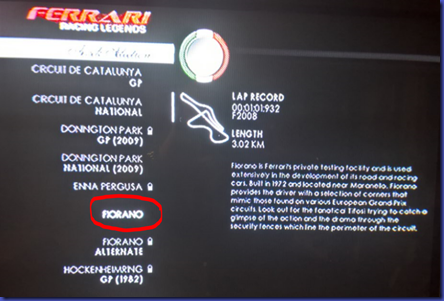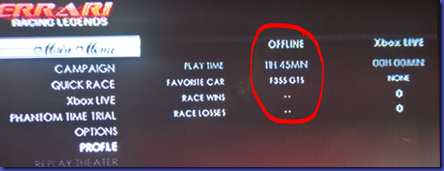# Weekly Price Guide–29 May 2016

F355 models (all models)

Average = \$179,600.00

Change = -\$3,600

360 (all models)

Average = \$139,248.80

Change = -\$139.50

F430 (all models)

Average = \$237,690.60

Change = -\$71.40

458 Italia

Average = \$414,485.00

Change = –\$7,291.00

458 Spider

Average = \$471,472.50

Change = -\$7,174.20

Value = (Avg price / cars)

458 Spider = \$78,578.75

458 Italia = \$41,448.50

F355 = \$35,120.00

F430 = \$14,855.66

360 = \$6,962.44

Brand value

Ferrari = \$25,393.07

Change = \$5,984.70 (20.25%)

# Weekly Price Guide–22 May 2016

F355 models (all models)

Average = \$179,200.00

Change = \$0

360 (all models)

Average = \$129,388.30

Change = -\$3,340.40

F430 (all models)

Average = \$237,762.00

Change = \$1,872.00

458 Italia

Average = \$421,776.00

Change = –\$10,938.40

458 Spider

Average = \$478,646.70

Change = -\$329.30

Value = (Avg price / cars)

458 Spider = \$59,830.84

458 Italia = \$35,148.00

F355 = \$29,866.67

F430 = \$14,860.13

360 = \$7,336.23

Brand value

Ferrari = \$29,408.37

Change = -\$4,035.76 (-12.07%)

# Weekly Price Guide–15 May 2016

F355 models (all models)

Average = \$179,200.00

Change = -\$1,400

360 (all models)

Average = \$142,728.70

Change = \$1,001.40

F430 (all models)

Average = \$235,890.00

Change = -\$2,743.00

458 Italia

Average = \$432,714.40

Change = \$5,313.70

458 Spider

Average = \$478,976.00

Change = -\$18,987.30

Value = (Avg price / cars)

458 Spider = \$62,425.14

458 Italia = \$43,271.44

F355 = \$29,866.67

F430 = \$18,145.38

360 = \$7,512.04

Brand value

Ferrari = \$33,444.13

Change = -\$1,195.83 (-3.45%)

# Fiorano unlockedMy upcoming Corsa Pilota is fast approaching and I have been toiling away on my Xbox working to unlock the Fiorano track so I can practice (virtually of course). As you can see by the above screen shot I have finally managed to do that.

Unfortunately, there no unlock cheats that I can find, so this has meant that I have had to work through all the challenges until I was finally able to unlock the Fiorano track.The above screen shot shows you the time that I invest to unlock just the Fiorano track (almost 12 hours of total play time).

The Ferrari Racing Legends Xbox game can be quite tough in some challenges and the controllability varies widely with the car in use (which kinda makes sense). This certainly makes it quite a challenging game and I can see why many people didn’t like the game much.

Thanks goodness I can now focus just on Fiorano and get my eye in before attempting the real thing soon!

# Weekly Price Guide–7 May 2016

F355 models (all models)

Average = \$180,600.00

Change = \$5,152.50

360 (all models)

Average = \$141,727.30

Change = \$858.80

F430 (all models)

Average = \$238,633.00

Change = -\$3,743.70

458 Italia

Average = \$427,400.70

Change = \$0

458 Spider

Average = \$497,963.30

Change = \$11,765.80

Value = (Avg price / cars)

458 Spider = \$62,245.41

458 Italia = \$47,488.97

F355 = \$36,120.00

F430 = \$19,886.08

360 = \$7,459.33

Brand value

Ferrari = \$34,639.96

Change = -\$1,185.20 (-3.31%)

# Weekly price Guide–1 May 2016

F355 models (all models)

Average = \$180,600.00

Change = \$0

360 (all models)

Average = \$140,868.50

Change = \$238.30

F430 (all models)

Average = \$242,376.70

Change = –\$761.30

458 Italia

Average = \$427,400.70

Change = \$3,764.90

458 Spider

Average = \$486,197.50

Change = -\$12,908.30

Value = (Avg price / cars)

458 Spider = \$81,032.92

458 Italia = \$47,488.97

F355 = \$36,120.00

F430 = \$22,034.25

360 = \$7,414.13

Brand value

Ferrari = \$38,818.05

Change = \$2,992.89 (+8.35%)